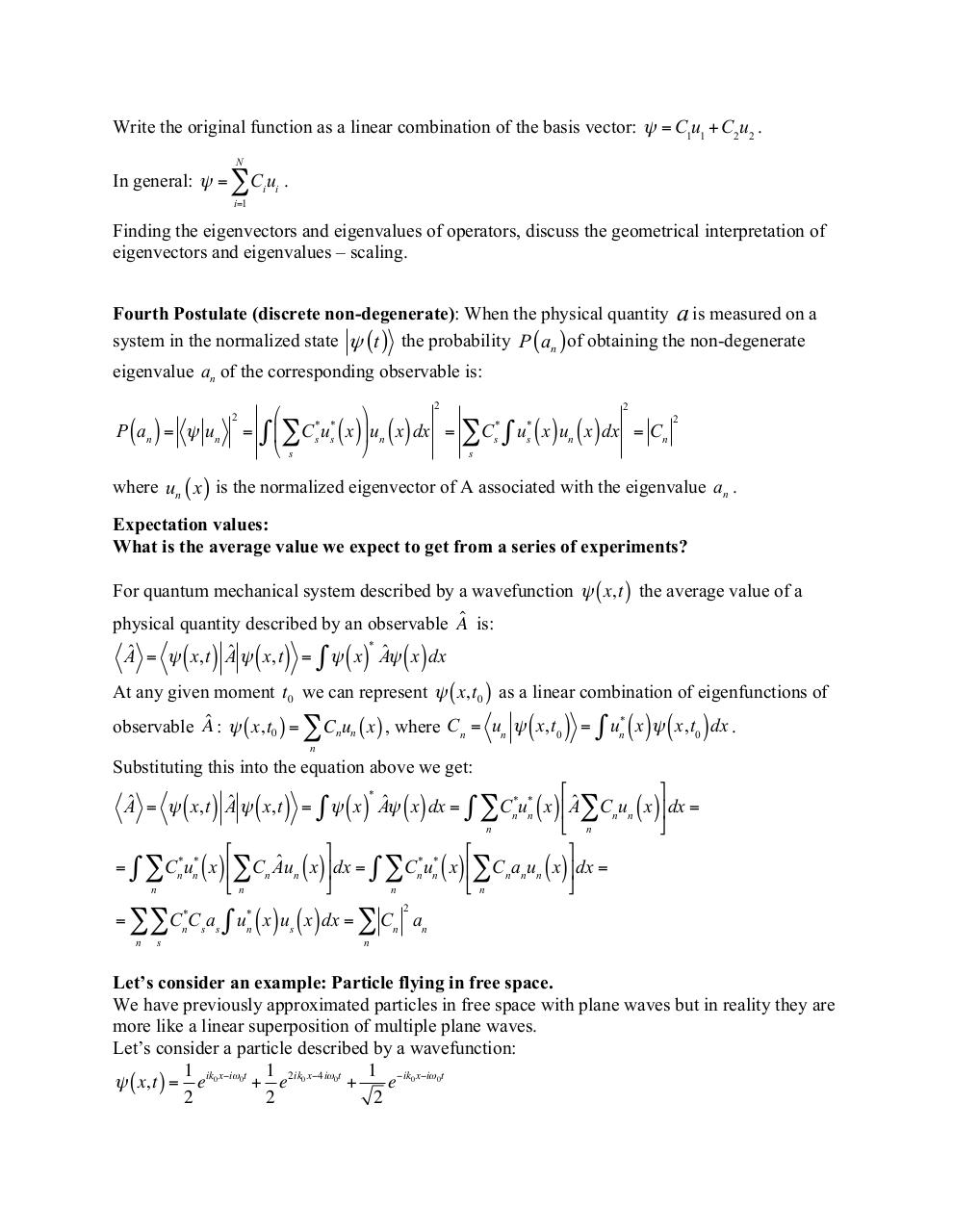# L7+QM+Measurements+and+Constants+of+Motion.pdfPage 1 2 3 4 5 6 7 8 9

#### Text preview

Write the original function as a linear combination of the basis vector: ψ = C1u1 + C2u2 .
N

In general: ψ = ∑Ci ui .
i=1

Finding the eigenvectors and eigenvalues of operators, discuss the geometrical interpretation of
eigenvectors and eigenvalues – scaling.
Fourth Postulate (discrete non-degenerate): When the physical quantity a is measured on a
system in the normalized state ψ (t ) the probability P ( an )of obtaining the non-degenerate
eigenvalue an of the corresponding observable is:
2

( )

P an = ψ un

2

&quot;
%
= ∫ \$∑Cs*us* x ' un x dx =
# s
&amp;

() ()

2
*
s

*
s

∑C ∫ u ( x) u ( x) dx
n

= Cn

2

s

where un ( x ) is the normalized eigenvector of A associated with the eigenvalue an .
Expectation values:
What is the average value we expect to get from a series of experiments?
For quantum mechanical system described by a wavefunction ψ ( x, t ) the average value of a
physical quantity described by an observable Aˆ is:
*
Aˆ = ψ x,t Aˆ ψ x,t = ∫ ψ x Aˆψ x dx

( )

( )

()

()

At any given moment t0 we can represent ψ ( x, t0 ) as a linear combination of eigenfunctions of
observable Aˆ : ψ ( x, t0 ) = ∑ Cn un ( x ) , where Cn = un ψ x,t0

*
n

( ) = ∫ u ( x)ψ ( x,t ) dx .

n

0

Substituting this into the equation above we get:

#
&amp;
* *
ˆ
C
u
x
A
C
u
x
%
∫ ∑ n n \$ ∑ n n (' dx =
n
n
#
&amp;
#
&amp;
ˆ x ( dx = ∫ ∑C *u* x %∑C a u x ( dx =
= ∫ ∑Cn*un* x %∑Cn Au
n
n n
n n n
\$n
'
\$n
'
n
n
*
Aˆ = ψ x,t Aˆ ψ x,t = ∫ ψ x Aˆψ x dx =

( )

()

( )

()

()

()

()

()

()

()

2

= ∑∑Cn*Cs as ∫ un* x us x dx = ∑ Cn an
n

s

() ()

n

Let’s consider an example: Particle flying in free space.
We have previously approximated particles in free space with plane waves but in reality they are
more like a linear superposition of multiple plane waves.
Let’s consider a particle described by a wavefunction:
1
1
1 −ik0 x−iω0t
ψ ( x, t ) = eik0 x−iω0t + e 2ik0 x−4iω0t +
e
2
2
2